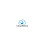### Python

• Don't repeat any code! If you do that, there is something wrong. Don't dry (don't repeat yourself)

It is a Case-Sensitive language. Keep your variable lower case letters. No space, If you need, use _ .  Ex: my_language

print ('Hello World')
print(f"{kind} kilos ")     # kind is a variable which defined before.

name= input (' What is your name? ')
str: All input are str (default). You need to convert them int or float to make calculation
float
int

' ' '
You can write whatever you want here

' ' '

word= "Python and Mr.G"

print (word[0:4])  ==> Pyth
0 is P.
4 is o. But the last one is excluded.

Examples:
print (word)     ==> t
print (word[-1])     ==> G
print (word[2:5])     ==> tho
print(word[1:-1])  ==> ython and Mr.

Function = Methods
message.upper()                   # to convert to uppercase
message.lower()                   # to convert to lowercase
message.title()                      # to capitalize the first letter of every word
message.find(‘p’)                 # returns the index of the first occurrence of p (or -1 if not found)
message.replace(‘p’, ‘q’)

Arithmetic Operations
+
-
*
/                   # returns a float
//                  # returns an int
%                # returns the remainder of division
**                # exponentiation - x ** y = x to the power of y

x=x+3      is same as           x+=3
x=x-3       is same as           x-=3

Import any module
If you want to import math module; just type import module_name
For Ex:
import math
Then call function like   math.function_name  ex: math.floor (2.8)  => result is 2.

*search python3 math module on Google to see more

IF STATEMENT
if                    # Don't forget to put : after if
elif                 # it means   else if
else                # when it catches if; it goes out of if statement

# Feel free to use "and" & "or& "and not& "if notto conditional usage. and&or must be lower case, not upper case!
# You can put another if in the if statement.

number = input ("put a number==> ")
b=int(number)

if b>300:
print("number is bigger 300")

elif b<200 and b>100:
print("number you entered is between 100 and 200")

else:
print("nooooooopeee")

Note: if start:    is equal to    if start == True:

Comparison operators
a > b
a >= b (greater than or equal to)
a < b
a <= b
a == b (equals)
a != b (not equals)

While LOOP
It is a loop.

while

break    # it breaks while loop. It means the code goes the end of the while loop. If you use break function in while you can use "while:True" as a statement. If you don't put break, it goes forever.

else             # else may be used for while loop as well!

for   LOOP
It is a loop.

`for a in (1,2,3):    print(a)`

`for a in range (1,10):    print(a)`

1.Actually quite glad to state, your post is fascinating to peruse. I never stop myself to say something regarding python. oracle fusion SCM training india